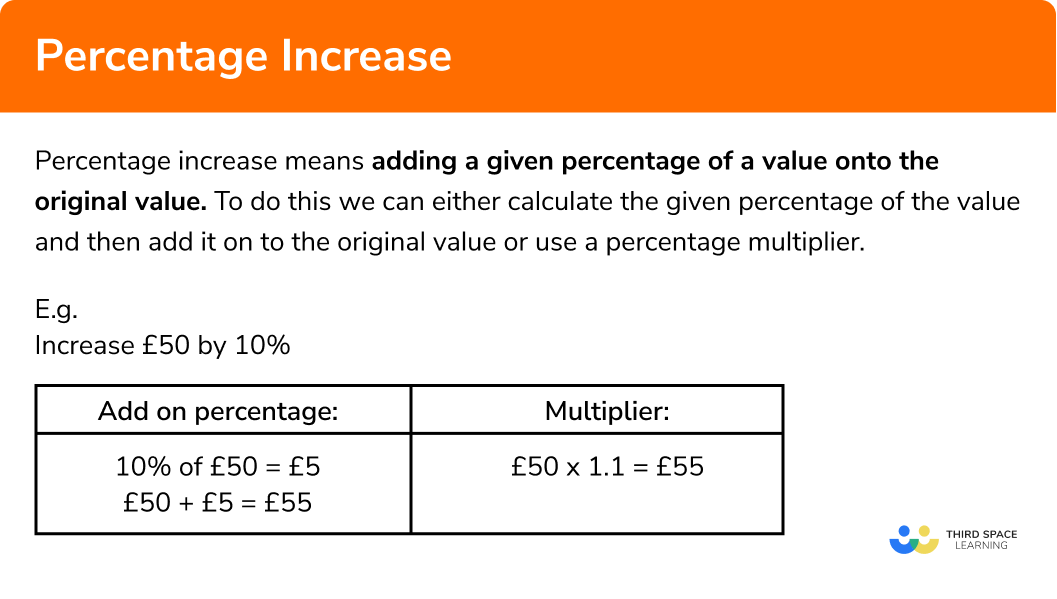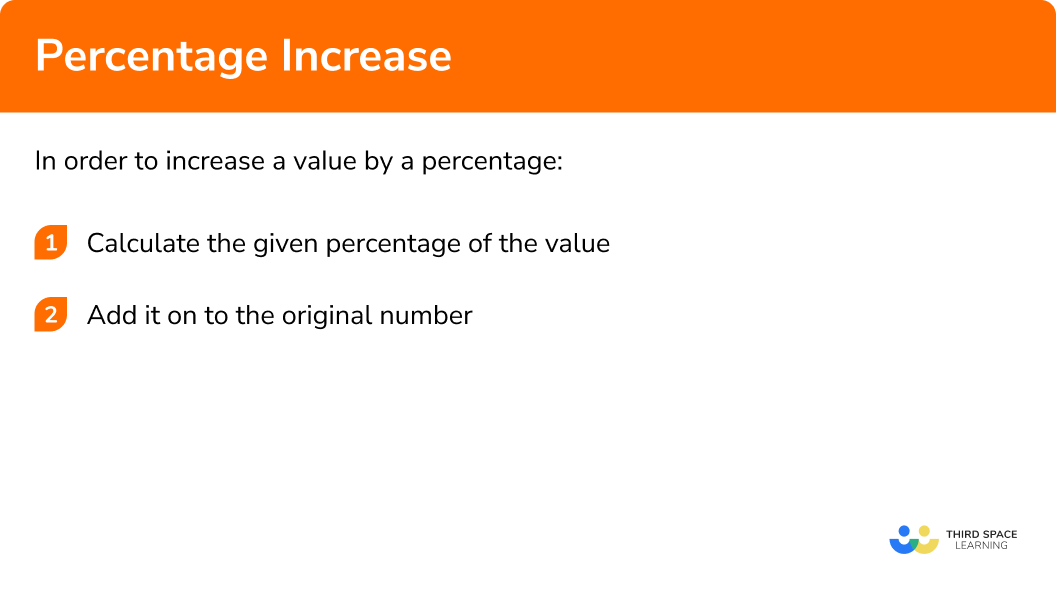GCSE Maths Number FDP Percentages

Percentage Increase

# Percentage Increase

Here is everything you need to know about percentage increase for GCSE maths (Edexcel, AQA and OCR). You’ll learn how to increase a value by a given percentage,  use multipliers to calculate percentage increase and work out percentage change.

Look out for the percentage increase and decrease worksheets and exam questions at the end.

## What is percentage increase?

Percentage increase means adding a given percentage of a value on to the original value. To do this we can either calculate the given percentage of the value and then add it on to the original value or use a percentage multiplier.

### Explain what percentage increase is?## How to increase a value by a percentage

In order to increase a value by a percentage:

1. Calculate the given percentage of the value.
2. Add it on to the original number.

### What are the 2 steps to increase a value by a percentage?## How to work out percentage increase examples

### Example 1: non-calculator

Increase £50 by 40%

1. Calculate 40% of £50

To do this without a calculator, the easiest way is to calculate 10% (by dividing by 10) and then multiply by 4 to get 40%.

10% of £50 = £5

40% of £50 = £20

2 Add £20 on to the original value

£50 + £20 = £70

### Example 2: non-calculator

Increase 400 by 65%

10% of 400 = 40

60% of 400 = 240

5% of 400 = 20

65% of 400 = 260

400 + 260 = 660

### Example 3: using a calculator

Increase 350m by 27%

When using a calculator to calculate a percentage, we divide the value by 100 to find 1% and then multiply by the percentage that we want.

\begin{array}{l} 350 \div 100=3.5 \\ 1\%=3.5\\\\ 3.5 \times 27=94.5 \mathrm{~m}\\ 27\%=94.5 \end{array}

350m + 94.5m = 444.5m

### Example 4: using a calculator

The price of Katrina’s train ticket last year was £72. This year it has increased by 17.5%. Find the price of Katrina’s train ticket this year.

$\begin{array}{l} 72 \div 100=0.72 \\ 1\%=0.72\\\\ 0.72 \times 17.5= £ \hspace{1mm} 12.60\\ 17.5\%= £ \hspace{1mm} 12.60 \end{array}$

£72 + £12.60 = £84.60

## How to increase a value by a percentage using a percentage multiplier

We can increase a value by a percentage using a percentage multiplier. A percentage multiplier is a decimal that is related to the percentage you are trying to find.

1. Add the percentage we are increasing by to 100%

We add it on to 100% because we are increasing the original value.

2Convert to a decimal

3Multiply the original amount by the decimal

### Example 5: using a multiplier

Increase 3200ml by 24%

$100\% + 24\% = 124\%$

$124\% = 1.24$

$3200\times 1.24=3968ml$

### Example 6: using a multiplier

In 2015 Rachel’s monthly rent was £620. Over the course of five years, Rachel’s monthly rent increased by 12.5%. Find Rachel’s monthly rent at the end of the five years.

$100\% + 12.5\% = 112.5\%$

$112.5\% = 1.125$

620 × 1.125 = £697.50

Remember to always write money using two decimal places.

## How to calculate percentage increase between two values

Given two values, we can calculate the percentage change. This may also be called percentage difference, percentage increase, percentage gain or percentage profit.

We can calculate percentage change using the percentage change formula:

$\text { Percentage change }=\frac{\text { Change }}{\text { Original }} \times 100$

The same formula can be used to calculate percentage decrease

1. Work out how much the value has changed by subtracting the old number from the new number.
2. Apply the percentage change formula

### Example 7: calculating percentage change

The weight of a lamb has increased from 4kg to 5.2kg. Find the percentage increase in the lamb’s weight.

$5.2 − 4 = 1.2kg$

\begin{array}{l} \text { Percentage change }&=\frac{\text { Change }}{\text { Original }} \times 100 \\\\ \text { Percentage change}&=\frac{1.2}{4} \times 100\end{array}

Percentage change = 30%

The lamb’s weight has increased by 30%.

### Example 8: calculating percentage profit

Lucy buys an antique table for £150 and sells it for £185. Calculate Lucy’s percentage profit.

£185 – £150 = £35

$\text { Percentage change }=\frac{35}{150} \times 100$

Percentage change = 23.3333333..%

The percentage profit is 23.3% to 1 decimal place.

### Common misconceptions

• Percentages greater than 100%

Believing that percentages cannot be greater than 100%. For percentage increase using multipliers we encounter a lot of percentages greater than 100% as we are adding on to the original 100%.

• Converting between percentages and decimals

Incorrectly converting percentages to decimals. The most common mistakes are with single digit percentages (e.g. 5%), multiples of 10 (e.g. 40%) and decimal percentages (e.g. 3.2%)

Remember to divide the percentage by 100 to find the decimal.

E.g.

5% = 0.05,

40% = 0.4,

3.2% = 0.032,

106.5% = 1.065

• Using an incorrect value for the denominator in the percentage increase formula

Using the new value instead of the original value for the denominator when calculating percentage change.

Percentage increase is part of our series of lessons to support revision on percentages. You may find it helpful to start with the main percentages lesson for a summary of what to expect, or use the step by step guides below for further detail on individual topics. Other lessons in this series include:

### Percentage increase practice questions

1. Increase £520 by 20\% .

£540£104£624£64020\% of 520 is 104 . This can be added to the original amount to find the quantity after the increase.

2. Increase 3420m by 43\% .

3463m4890.6m1470.6m3563m43\% of 3420 is 1470.6 . This can be added to the original amount to find the quantity after the increase.

3. Use a multiplier to increase 38 by 14\% .

5.3243.324233.3The multiplier we need to use is 1.14 , so the calculation is 1.14\times38 .

4. Use a multiplier to increase 650kg by 26.5\% .

172.25kg822.25kg513.83kg676.5kgThe multiplier we need to use is 1.265 , so the calculation is 1.265\times650 .

5. Find the percentage increase when 400ml is increased to 620ml .

55\%155\%220\%110\%The amount has increased by 220ml . As a percentage of the original amount, this is  \frac{220}{400}\times100 .

6. Find the percentage profit when Karam buys a house for £124000 and sells the house for £137640 .

111\%89\%11\%136.4\%The percentage profit is calculated as \frac{137640-124000}{124000}\times100 .

### Percentage increase GCSE questions

1. (a) Catrin’s salary is £18000 . She receives a 3.5\% pay rise.

Work out Catrin’s new salary.

(b) How much extra money will Catrin earn each week?

(3 marks)

(a)

3.5\% of  \pounds 18000 = \pounds 630

(1)

\pounds 18000 + \pounds 630 = \pounds 18630

(1)

(b)

\pounds 630 \div 52 = \pounds 12.12

(1)

2. In 2010 the population of the UK was approximately 62220000 .

By 2020 it had increased to 66520000 .

Find the percentage increase in population over these 10 years.

(2 marks)

66520000 – 62220000 = 4300000

(1)

\frac{4300000}{62220000} \times 100=6.91\%

(1)

3. Thomas bought a box containing 100 packets of crisps for £30 .

He sold 96 packets of crisps for 50p each.

Calculate Thomas’s percentage profit.

(3 marks)

96 \times 50 p = \pounds 48

(1)

\pounds 48-\pounds 30=\pounds 18

(1)

\frac{18}{30} \times 100=60 \%

(1)

## Learning checklist

You have now learned how to:

• Increase a value by a given percentage
• Use a percentage multiplier to increase a value by a percentage
• Calculate percent increase between two values

## Still stuck?

Prepare your KS4 students for maths GCSEs success with Third Space Learning. Weekly online one to one GCSE maths revision lessons delivered by expert maths tutors.

Find out more about our GCSE maths tuition programme.